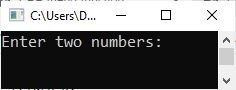# C++ friend function

In this post, you will learn everything there is to know about the friend function in C++, including an example program and a step-by-step explanation of the code.

## What exactly is the friend function?

A friend function is a class function that has the same access as a class's member functions. The friend function, however, is not a member function. If the keyword "friend" is used before any function declared within the class, that function will be referred to as the "friend" function.

## Where is the friend function declared?

Inside the class where a member function of the same class is declared, a friend function is declared. However, the friend function is not a member of the class to which it belongs (or where it is declared).

To declare a function as a friend function, use the friend keyword. Alternatively, use this keyword before the function name. As an example,

`friend void myFun();`

The friend function is defined outside the class (where it is declared). To define a friend function, the friend keyword is not required. This keyword is required only while declaring the function inside the class.

## Why is the keyword "friend" used?

It is used to tell the compiler that it is not a member function of the class. Rather, it is a friend function. Because if you remove the keyword "friend," then this function gets processed by the compiler as a member function of the class.

## How to call the "Friend" function

The friend function cannot be called on any object, or the friend function has no caller object. because it is not a member function of the class where it is declared. And, when defining the function, there is no membership label attached. Therefore, to call this function from the main() function, just call it as a normal function call.

## Why would you use the friend function?

In a big project, there are a lot of programmers who work for the same project. Therefore, using the friend function, one programmer can access the private data of members of the class defined by another programmer.

For example, suppose a programmer named James wants to access the private data of a member of a class that is defined by another programmer named Ricky. So, Ricky declares a friend function inside the class defined by him and tells the name of this function to his friend James, who works for the same project. Now James can use this function to access private data for members of the class defined by Ricky.

## Step-by-Step Explanation of the C++ Friend Function

Now let's take an example that multiplies any two numbers entered by the user. There is a friend function in this program. Let's have a look at the program; later, I'll explain each and everything that goes inside the program in a step-by-step manner.

```#include<iostream>
using namespace std;

class CodesCracker
{
private:
int m, n, res;
public:
void getData()
{
cout<<"Enter two numbers: ";
cin>>m>>n;
}
void showResult()
{
cout<<"\nResult = "<<res;
}
friend void mulFun(CodesCracker &c);
};
int main()
{
CodesCracker ob;
ob.getData();
mulFun(ob);
ob.showResult();
cout<<endl;
return 0;
}
void mulFun(CodesCracker &x)
{
x.res = (x.m) * (x.n);
}```

This program was built and runs under the Code::Blocks IDE. The snapshot given below shows a sample run of the above program:Now supply two numbers, say 5 and 6. Press the ENTER key to see the output as shown in the following snapshot:Here is a step-by-step explanation of the previous program:

• Program flow always starts with the main() function.
• Therefore, in the main() function, there is a statement
`CodesCracker ob;`
which tells the compiler to create an object "ob" of the class CodesCracker.
• The compiler, however, looks for the CodesCracker class before creating an object. That is, whether it is defined or not.
• Because it is defined, then an object named "ob" gets created.
• Using this object "ob," a member function getData() gets called.
• Because "ob" is the object declared for the class CodesCracker, function getData() from this class only gets called.
• This function asks the user to enter any two numbers and stores them in the "m" and "n" variables.
• These variables are declared as private data members of the same class.
• Now a friend function called mulFun() gets called with "ob" as its parameter.
• So the program checked whether or not this function was declared within the class of that object.
• Because it is declared inside the same class as the "friend" keyword.
• So this function is a friend to that class.
• A friend of the same class means it can access the private data of that class.
• Because it is already declared in the same class as that object, it is passed as its parameter.
• So the program goes to the function definition part.
• In the function definition, the statement
`x.res = (x.m) * (x.n);`
defines that the value of the m and n variables of the class to which the object "ob" is declared gets multiplied and stored in a variable res of the same class.
• Now the program flow again goes back to the main() function.
• And the statement ob.showResult() gets executed, where the value of the res variable gets printed on the output screen.

C++ Quiz

« Previous Tutorial Next Tutorial »